# ISC Class 12 Maths Important Questions

The ISC Class 12 Maths Important Questions are prepared to keep in mind the need to give an overview of the major topics and concepts under the syllabus of ISC Class 12the students. Maths is considered to be a tough subject, and it requires a lot of practice to excel in the subject. The ISC Class 12 important questions prepared as per the Maths Syllabus can help students to get the practice they need. Your problem-solving skills will undoubtedly, get better with practice. The questions compiled here are done after analyzing the questions that are often asked in the exams.

### The Important Questions of ISC Class 12 Maths are given below:

1. A binary operation * defined on Q-{1} is given by a * b = a+b – ab. Find the identity element.
2. A family has two children. What is the probability that both children are boys, given that at least one of them is a boy?
3. Let R+ be the set of all positive real numbers and f: R+ [4, ∞ ): f(x) = + 4. Show that inverse of f exists and find
$$\begin{array}{l}f^{-1}\end{array}$$
.
4. A circular disc of radius 3 cm. is heated. Due to expansion, its radius increases at the rate of 0•05 cm/s. Find the rate at which its area increases when the radius is 3.2 cm.
5. An open-topped box is to be made by removing equal squares from each corner of a 3 m by 8 m rectangle sheet of aluminium and by folding up the sides. Find the volume of the largest such box.
6. A, B and C throw a die one after the other in the same order till one of them gets a ‘6’ and wins the game. Find their respective probability of winning, if A starts the game.
7. Find the cost of increasing from 100 to 200 units if the marginal cost in Rupees per unit is given by the function MC = 0 003 x2– 0 01 x + 2 5.
8. If two lines of regression are 4x + 2y – 3=0 and 3x +6y +5 =0, find the correlation coefficient between x and y.
9. Given that the observations are (9,-4), (10, -3), (11,-1), (13,1), (14,3), (15,5), (16,8), find the two lines of regression. Estimate the value of y when x = 13.5.
10. Find the regression coefficient and the two lines of regression for the following data.
 X 2 6 4 7 5 Y 8 8 5 6 2

Also, compute the correlation coefficient.

11. A toy company manufactures two types of dolls, A and B. Market tests and available resources have indicated that the combined production level should not exceed 1200 dolls per week, and the demand for the dolls of type B is atmost half of that for dolls of type A. Further, the production level of type A can exceed three times the production of dolls of other types by at most 600 units. If the company makes a profit of Rs12 and Rs16 per doll, respectively, on dolls A and B, how many of each type of doll should be produced weekly in order to maximize the profit?
12. Find
$$\begin{array}{l}\frac{dy}{dx}\end{array}$$
, if
$$\begin{array}{l}x=at^{2}\end{array}$$
and
$$\begin{array}{l}y=2at\end{array}$$
13. Find the differential equation of the family of curves
$$\begin{array}{l}y=Ae^{x}+Be^{-x}\end{array}$$
, where A and B are arbitrary constants.
14. Find the intervals in which the function f(x) is strictly increasing where,
$$\begin{array}{l}f(x)=10-6x-2x^{2}\end{array}$$
15. Prove that the function f(x) =|x-1|, xR is continuous at x = 1 but not differentiable.
16. Evaluate: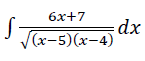17. Prove that: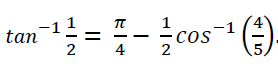18. Verify Rolle’s Theorem for the following function: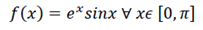• Solve the following differential equation: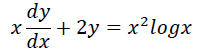• Let X denote the number of hours you study during a randomly selected school day. The probability that X can take the value ‘x’ has the following form, where ‘k’ is some unknown constant.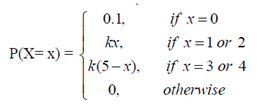1. Find the value of ‘k’.
2. What is the probability that you study:
1. at least two hours?
2. exactly two hours?
3. at most 2 hours?